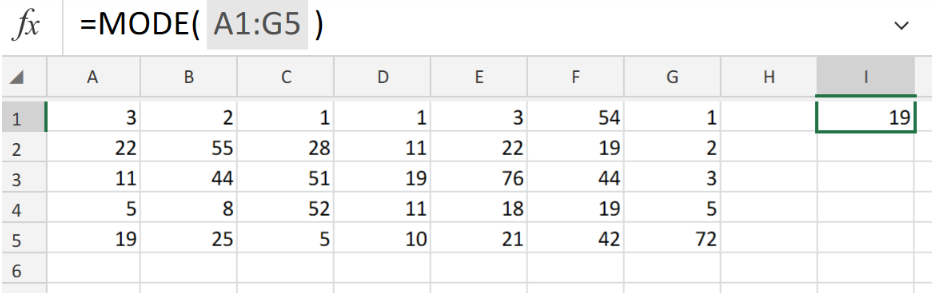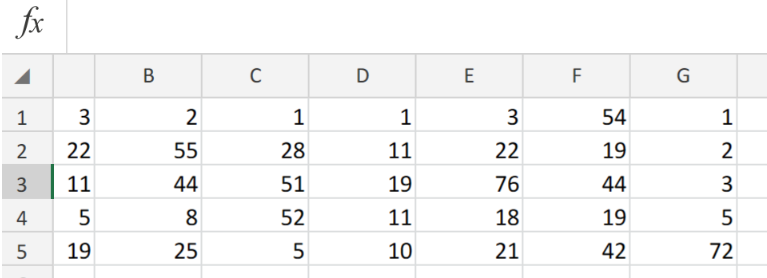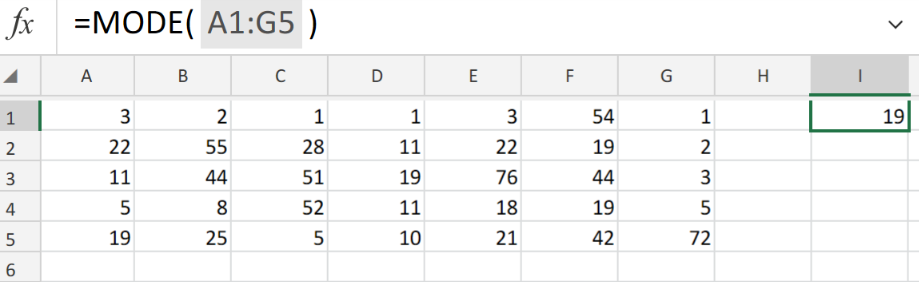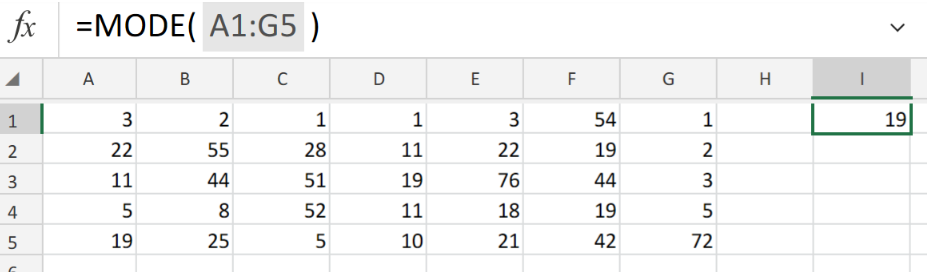Get instant live expert help with Excel or Google Sheets“My Excelchat expert helped me in less than 20 minutes, saving me what would have been 5 hours of work!”

#### Post your problem and you’ll get Expert help in seconds.

Your message must be at least 40 characters
Our professional Expert are available now. Your privacy is guaranteed.

# Excel MODE FunctionFigure 1. of Excel Mode Function.

Let’s say we are required to find the most commonly occurring number among a range of cells in our worksheet. We are going to utilize the MODE Function in Excel.

## Generic Formula

`=MODE (number1, [number2], ...)`

Where number1 = the first number value within our range of cells.

number2 (optional) = the next number value within our cell range.

## How to use the Excel MODE Function.

Excel MODE function will determine a central tendency of an range of numbers. The main objective being to calculate the most frequently occurring figure. We can achieve this by following two simple steps!

1. Collect the number values available to us and arrange them in our worksheet.Figure 2. of Number Values in Excel.

1. The formula that we are going to enter into cell I1 to calculate the mode of the number range above is as follows;

`=MODE(A1:G5)`Figure 3. of Mode Function in Excel.

This will instruct Excel to look through our range of cells and determine the most frequently occurring number value (19).

The MODE function is an inbuilt Excel Function which is commonly categorized as a Statistical operation. The MODE Function determines the most regularly occurring, or incessant, element in a variety or an array of data.Figure 4. of Final Result.

## Instant Connection to an Expert through our Excelchat Service

Our live Excelchat Service is here for you. We have Excel Experts available 24/7 to answer any Excel questions you may have. Guaranteed connection within 30 seconds and a customized solution for you within 20 minutes.

### Did this post not answer your question? Get a solution from connecting with the expert.Another blog reader asked this question today on Excelchat:## Subscribe to Excelchat.coAnother blog reader asked this question today on Excelchat: